Korbit’s Data Science Learning Modules

Korbit offers everything you need from A-Z about data science in one place.
See our comprehensive list of learning modules below.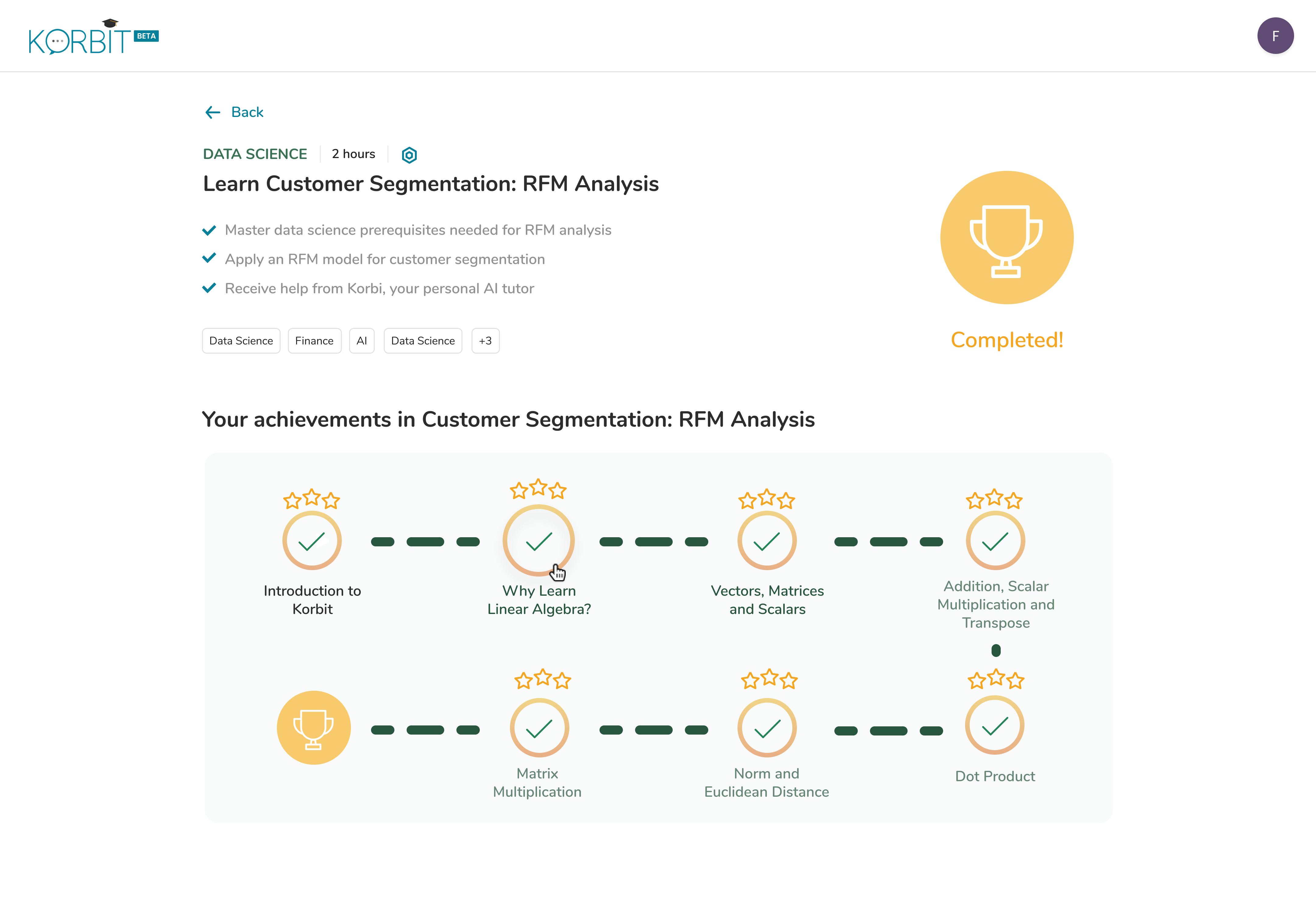Comprehensive Course Catalogue

Explore Korbit’s learning modules and projectsKorbit Platform

1. Tutorial

Welcome to Korbit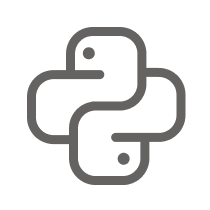Python

2. Hello Python

- Hello Python
- Variables and Types
- Operators and Expressions
- Input / Output
- If Else
- For Loops
- While LoopsMathematics

3. Probability Basics

- Introduction to Probability
- Discrete vs. Continuous Random Variables
- Expected Value vs. Sample Mean
- Variance and Standard Deviation
- Binomial Distribution
- Normal Distribution
- Joint and Marginal Probability
- Distributions
- Conditional Probability
- Bayes' Theorem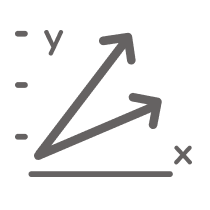Mathematics

4. Linear Algebra Basics

- Why Learn Linear Algebra?
- Vectors, Matrices and Scalars
- Multiplication and Transpose
- Dot Product
- Norm and Euclidean Distance
- Matrix Multiplication
- Identity MatrixStatistics

5. Introduction to Statistics

- Introduction to Statistics
- Levels of Measurement and Sampling Techniques
- Graphs
- Mean, Median and Mode
- Percentiles and QuartilesData science

6. Exploratory Data Analysis

- Descriptive Statistics
- Introduction to Graphs
- What is a Dataset?
- Exploratory Data AnalysisData science

7. RFM Analysis for Customer Segmentation

- Descriptive Statistics
- Introduction to Graphs
- What is a Dataset?
- Exploratory Data Analysis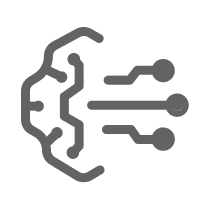Machine learning

8. What is Machine Learning?

- What is Machine Learning?
- Supervised Learning
- Classification
- Regression
- Unsupervised Learning
- Clustering
- Reinforcement Learning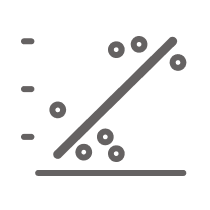Machine learning

9. Linear Regression

- Linear Regression
- Interpolation vs. Extrapolation
- Evaluation Metrics (Regression)
- Linear Regression with Categorical Features
- Conditions for Linear Regression
- Handling Outliers in Linear Regression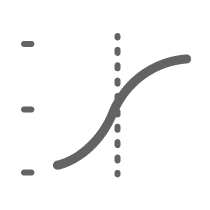Machine learning

10. Logistic Regression

- What is Machine Learning?
- Classification
- Binary Classification
- Logistic Regression Basic
- Sigmoid Function
- Evaluation Metrics (Classification)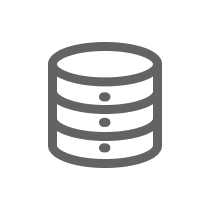Machine learning

11. Data Preprocessing

- Overview of Data Preprocessing
- Data Cleaning
- Handling Outliers
- Splitting Data
- Feature Engineering
- One-Hot Encoding
- Feature Importance
- Feature Scaling
- Dimensionality Reduction
- Feature Selection
- Principal Component AnalysisMachine learning

12. Classification

- Classification
- Binary Classification
- Logistic Regression Basic
- Sigmoid Function
- Evaluation Metrics (Classification)
- Binary Classification for Imbalanced Classes
- Naive Bayes’ Classifiers
- K-Nearest NeighboursMachine learning

13. Foundational Machine Learning Theory

- Splitting Data
- Cost and Loss Functions
- Cross Validation
- Parameters vs. Hyperparameters
- Hyperparameter Tuning
- Overview of Regularization
- L1 vs. L2 Regularization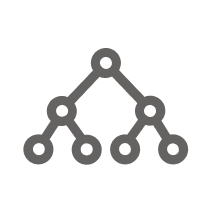Machine learning

14. CART Decision Trees and Random Forests

- Introduction to Decision Trees
- CART Decision Tree Splits
- Decision Tree Selection Criteria
- Introduction to Random ForestsMachine learning

15. Unsupervised Learning

- Unsupervised Learning
- Clustering
- K-Means Clustering
- Dimensionality Reduction
- Principal Component AnalysisMachine learning

16. Supervised Learning

- Motivation
- Supervised Learning
- Linear Approximators
- Generalized Linear Approximators
- Overfitting and Underfitting
- Bias and Variance: Cross-Validation
- Bias-Variance Decomposition
- Overview of Logistic Regression
- Regularization for Logistic RegressionMachine learning

17. Predicting Credit Card Fraud

- What is a Dataset?
- Exploratory Data Analysis
- Splitting Data
- Feature Scaling
- Principal Component Analysis
- Logistic Regression Basic
- Evaluation Metrics (Classification)
- Predicting Credit Card Fraud with Logistic RegressionMachine learning

18. Predictive Maintenance

- Introduction to Decision Trees
- CART Decision Tree Splits
- Decision Tree Selection Criteria
- Introduction to Random Forests
- Overview of Data Preprocessing
- Feature Engineering
- Feature Scaling
- Feature Selection
- Splitting Data
- Cross Validation
- Hyperparameter Tuning
- Predictive MaintenanceMachine learning

- Exploratory Data Analysis
- Overview of Data Preprocessing
- One-Hot Encoding
- Feature Importance
- Feature Scaling
- Feature Selection
- Logistic Regression Basic
- Evaluation Metrics (Classification)Machine learning

20. Handling Outliers

- Introduction to Graphs
- Data Cleaning
- Handling Outliers
- Handling Outliers in Linear RegressionMachine learning

21. Feature Manipulation

- Feature Engineering
- One-Hot Encoding
- Feature Scaling
- Dimensionality Reduction
- Feature Importance
- Feature Selection
- Principal Component Analysis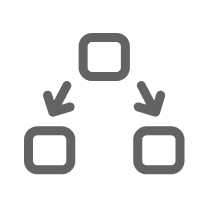Machine learning

22. Splitting Data

- What is a Dataset?
- Splitting Data
- Cross ValidationMachine learning

23. Regularization

- Splitting Data
- Cost and Loss Functions
- Overview of Regularization
- L1 vs. L2 RegularizationMachine learning

24. Dimensionality Reduction

- Unsupervised Learning
- Dimensionality Reduction
- Feature Scaling
- Feature Selection
- Principal Component AnalysisMachine learning

25. Clustering

- Unsupervised Learning
- Clustering
- K-Means Clustering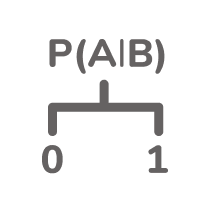Machine learning

26. Naive Bayes’ Classifiers

- Classification
- Evaluation Metrics (Classification)
- Joint and Marginal Probability Distributions
- Conditional Probability
- Bayes' Theorem
- Naive Bayes’ ClassifiersMachine learning

27. Hyperparameter Tuning

- Splitting Data
- Cost and Loss Functions
- Cross Validation
- Parameters vs. Hyperparameters
- Hyperparameter Tuning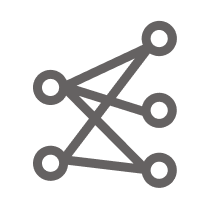Deep learning

28. Introduction to Neural Networks

- The Rise of Deep Learning
- An Artificial Neuron
- Example: 'OR' Neuron Using Sigmoid Activation
- Example: Neuron with Rectified Linear Function
- One-Layer Neural Network
- Example: 'XOR' Neural Network
- Deep Training Neural NetworksDeep learning

29. Training Neural Networks

- The Backpropagation Algorithm
- Optimization Difficulties
- Optimization Algorithms
- Visualizing How a Neural Network Is Trained
- Example: Data Preprocessing
- Overview of Model SelectionDeep learning